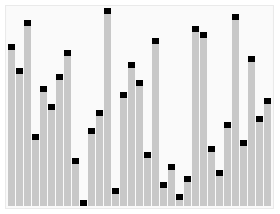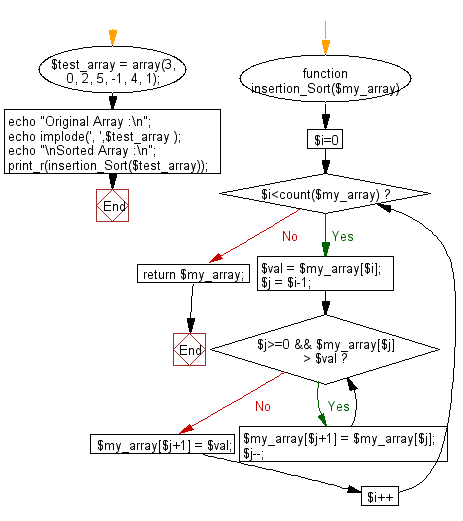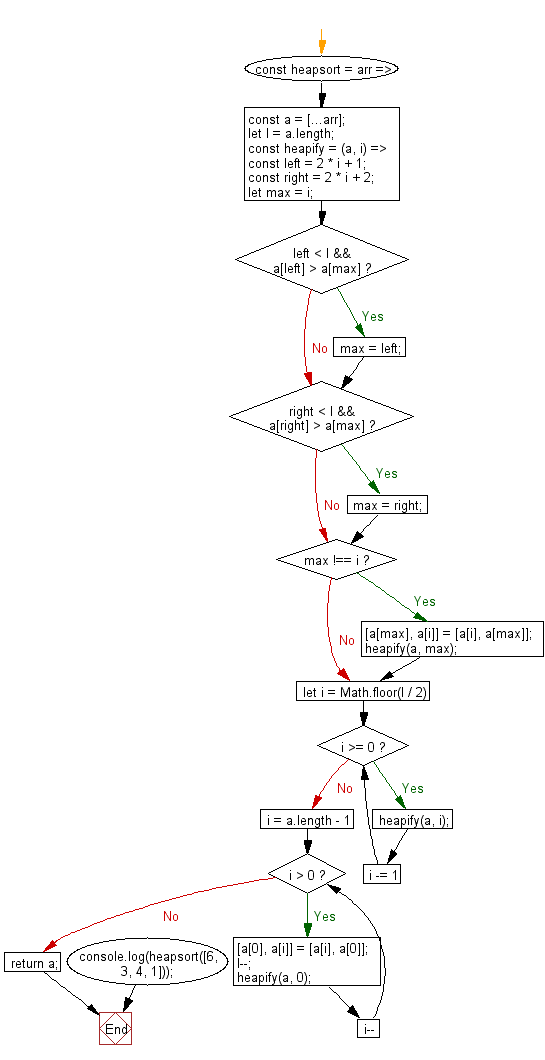# JavaScript Sorting Algorithm: Sorts an array of numbers, using the heapsort algorithm

## JavaScript Sorting Algorithm: Exercise-3 with Solution

Write a JavaScript program to sort a list of elements using Heap sort.

In computer science, heapsort (invented by J. W. J. Williams in 1964) is a comparison-based sorting algorithm. Heapsort can be thought of as an improved selection sort: like that algorithm, it divides its input into a sorted and an unsorted region, and it interactively shrinks the unsorted region by extracting the largest element and moving that to the sorted region. The improvement consists of the use of a heap data structure rather than a linear-time search to find the maximum. Although somewhat slower in practice on most machines than a well-implemented quicksort, it has the advantage of a more favorable worst-case O(n log n) runtime. Heapsort is an in-place algorithm, but it is not a stable sort.

A run of the heapsort algorithm sorting an array of randomly permuted values. In the first stage of the algorithm the array elements are reordered to satisfy the heap property. Before the actual sorting takes place, the heap tree structure is shown briefly for illustration.Animation credits : RolandH

Sample Solution-1:

JavaScript Code:

``````
var array_length;
/* to create MAX  array */
function heap_root(input, i) {
var left = 2 * i + 1;
var right = 2 * i + 2;
var max = i;

if (left < array_length && input[left] > input[max]) {
max = left;
}

if (right < array_length && input[right] > input[max])     {
max = right;
}

if (max != i) {
swap(input, i, max);
heap_root(input, max);
}
}

function swap(input, index_A, index_B) {
var temp = input[index_A];

input[index_A] = input[index_B];
input[index_B] = temp;
}

function heapSort(input) {

array_length = input.length;

for (var i = Math.floor(array_length / 2); i >= 0; i -= 1)      {
heap_root(input, i);
}

for (i = input.length - 1; i > 0; i--) {
swap(input, 0, i);
array_length--;

heap_root(input, 0);
}
}

var arr = [3, 0, 2, 5, -1, 4, 1];
heapSort(arr);
console.log(arr);
```
```

Sample Output:

```[-1,0,1,2,3,4,5]
```

Flowchart:Sample Solution-2:

• Use recursion.
• Use the spread operator (...) to clone the original array, arr.
• Use closures to declare a variable, l, and a function heapify.
• Use a for loop and Math.floor() in combination with heapify to create a max heap from the array.
• Use a for loop to repeatedly narrow down the considered range, using heapify and swapping values as necessary in order to sort the cloned array.

JavaScript Code:

``````//Source:https://bit.ly/3hEZdCl
//HeapSort
const heapsort = arr => {
const a = [...arr];
let l = a.length;

const heapify = (a, i) => {
const left = 2 * i + 1;
const right = 2 * i + 2;
let max = i;
if (left < l && a[left] > a[max]) max = left;
if (right < l && a[right] > a[max]) max = right;
if (max !== i) {
[a[max], a[i]] = [a[i], a[max]];
heapify(a, max);
}
};

for (let i = Math.floor(l / 2); i >= 0; i -= 1) heapify(a, i);
for (i = a.length - 1; i > 0; i--) {
[a, a[i]] = [a[i], a];
l--;
heapify(a, 0);
}
return a;
};

console.log(heapsort([6, 3, 4, 1]));
```
```

Sample Output:

```[1,3,4,6]
```

Flowchart:Live Demo:

See the Pen searching-and-sorting-algorithm-exercise-3 by w3resource (@w3resource) on CodePen.

Improve this sample solution and post your code through Disqus

What is the difficulty level of this exercise?

Test your Programming skills with w3resource's quiz.

﻿